Group 1 --Highly Sensitive to Pollution:      Stonefly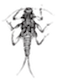Dobsonfly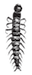Group 2 --Semi-Sensitive to Pollution:      Caddisfly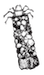Dragonfly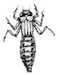Waterpenny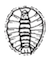Crawfish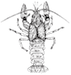Cranefly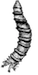Damselfly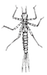Mayfly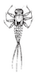Group 3 -- Semi-Tolerant of Pollution:      Orb Snail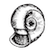Scud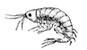Group 4 -- Tolerant of Pollution:      Sowbug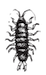Leech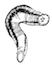Tubefix worm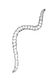Pouch Snail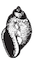You have just dredged a specified area of the streambed using a kicknet :)    Carefully drag the leaves and rocks away to reveal macroinvertebrates. Using the guide above, segregate macros into different types near the margins of the kicknet. Count the number of types (not number of individuals) per pollution tolerance Group, then submit the raw data and simple calculated values into the table below to determine a snapshot of overall stream health.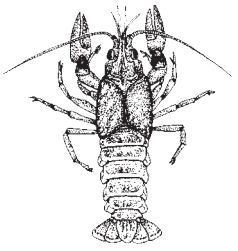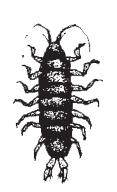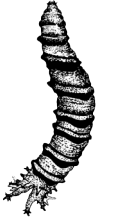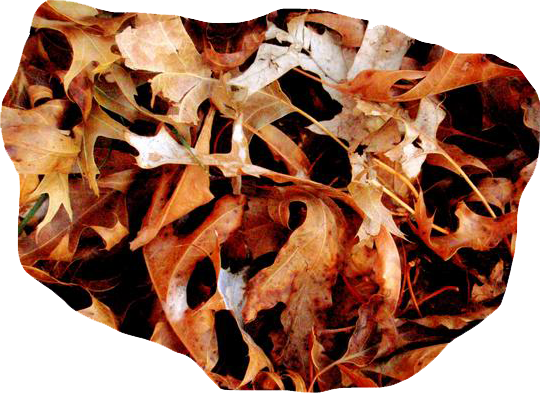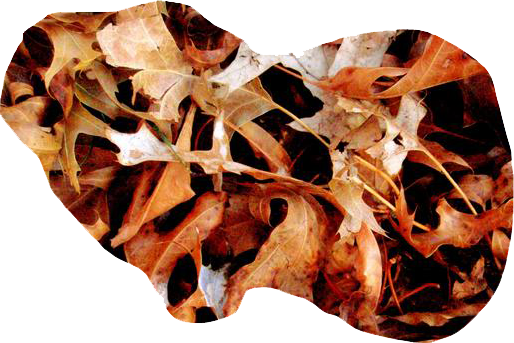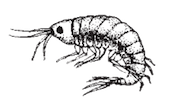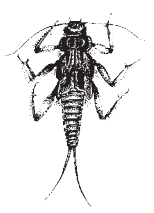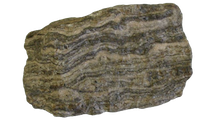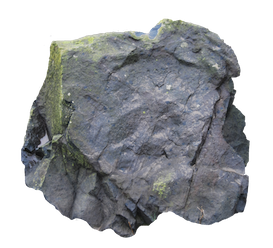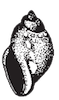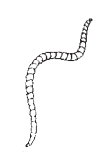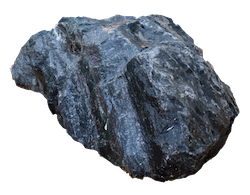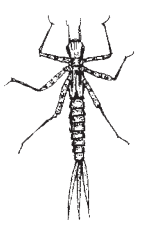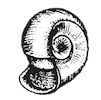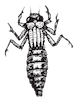Group 1: Types = (a) x 4 = (b) Group 2: Types = (a) x 3 = (b) Group 3: Types = (a) x 2 = (b) Group 4: Types = (a) x 1 = (b) Total Types (sum of a) = Total Value (sum of b) = Total Value (b) ÷ Total Types (a) =